# SSAT Upper Level Math : How to express a fraction as a ratio

## Example Questions

2 Next →

### Example Question #11 : How To Express A Fraction As A Ratio

Express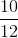as a ratio in simplest terms.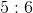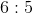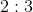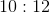Explanation:

First, reduce the fraction.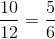Now, the simplified fraction can also be expressed as.

### Example Question #12 : How To Express A Fraction As A Ratio

A pan of cupcakes hadcupcakes on Monday. After one day,cupcakes were eaten. What is the ratio of cupcakes eaten to cupcakes remaining?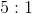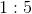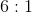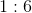Explanation:

First, find the number of cupcakes that remain.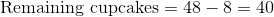Now, set up the ratio of cupcakes eaten to cupcakes remaining as a fraction: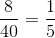That fraction is equivalent to.

### Example Question #13 : How To Express A Fraction As A Ratio

In a group of dolphins, there aremale dolphins for everyfemale dolphins. What is the ratio of female dolphins to male dolphins in this group?Explanation:

The ratio of female to male dolphins can be expressed as the following fraction: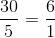That fraction can be expressed using the colon form of a ratio as.

### Example Question #14 : How To Express A Fraction As A Ratio

In an animal shelter, there arecats,birds, anddogs. What is the ratio of dogs to the total number of animals at the shelter?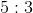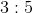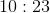Explanation:

First, find the total number of animals at the shelter.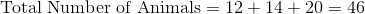The ratio of dogs to the total number of animals can be expressed as the following fraction: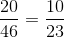That fraction can also be expressed as.

### Example Question #15 : How To Express A Fraction As A Ratio

There aremarbles in a bag. In the bag, there arered marbles,blue marbles, and the rest of the marbles are yellow. What is the ratio of yellow marbles to red marbles?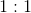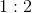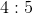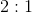Explanation:

First, find the number of yellow marbles in the bag.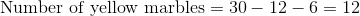The ratio of yellow marbles to red marbles can be expressed as a fraction: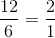That fraction can then be expressed as.

### Example Question #16 : How To Express A Fraction As A Ratio

A video store lent outvideos during the fall semester.of the videos were lost, and the rest were returned. What is the ratio of lost videos to returned videos?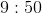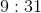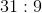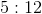Explanation:

First, find the number of videos that were returned.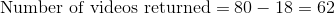Now, the ratio of videos lost to videos returned can be expressed as the following fraction: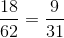This fraction can then be expressed as.

2 Next →